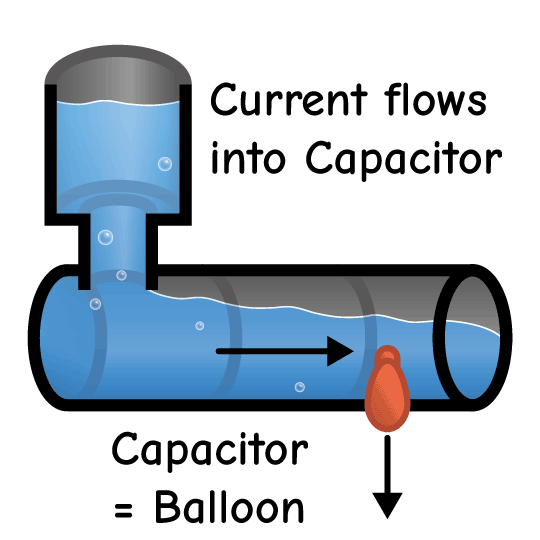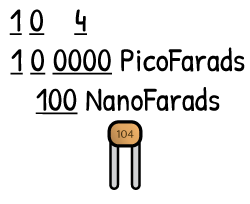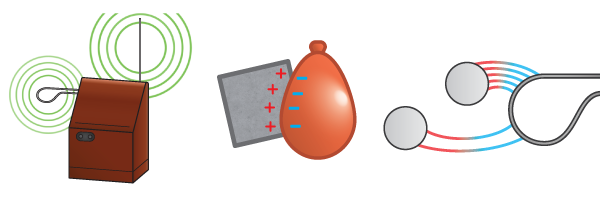# What's a Capacitor?A capacitor works like a “water balloon” connected to a water pipe. It stores up charge when a voltage is applied, the same way a balloon stores water under pressure.A water balloon stops filling when the pressure in the balloon equals the pressure in the pipe. And current flows into a capacitor until the voltage inside the capacitor equals the voltage it connects to.Inside a capacitor are two plates. These plates do not touch but are very close together. When voltage is applied, an electric field builds up between the plates. More charge builds up when the plates are larger or placed closer together, increasing capacitance.A bigger water balloon will store more water before it starts to push back, and a bigger capacitor will store more charge before voltage rises. Capacitance (C) is equal to the amount of charge (Q) stored per volt (V). This is like how a balloon’s capacity could be defined as the amount of water it can store for a given pressure. Capacitance is measured in farads, but common capacitors have values of nanofarads or picofarads. Nano means one billionth and pico means one trillionth. A 100 nanofarad capacitor would store 100 billionths of a coulomb of charge at one volt.Capacitors usually have a three digit number printed on them. Like with resistors, the third digit is how many zeros follow the first two digits. The code “104” means 10 followed by four zeros, or 100,000. This code is in units of picofarads, and 1000 picofarads is equal to 1 nanofarad. 100,000 divided by 1000 is 100, so we know this capacitor is 100 nanofarads.

### Click on an Article Below to Keep Learning